Ex 8.1

Serial order wise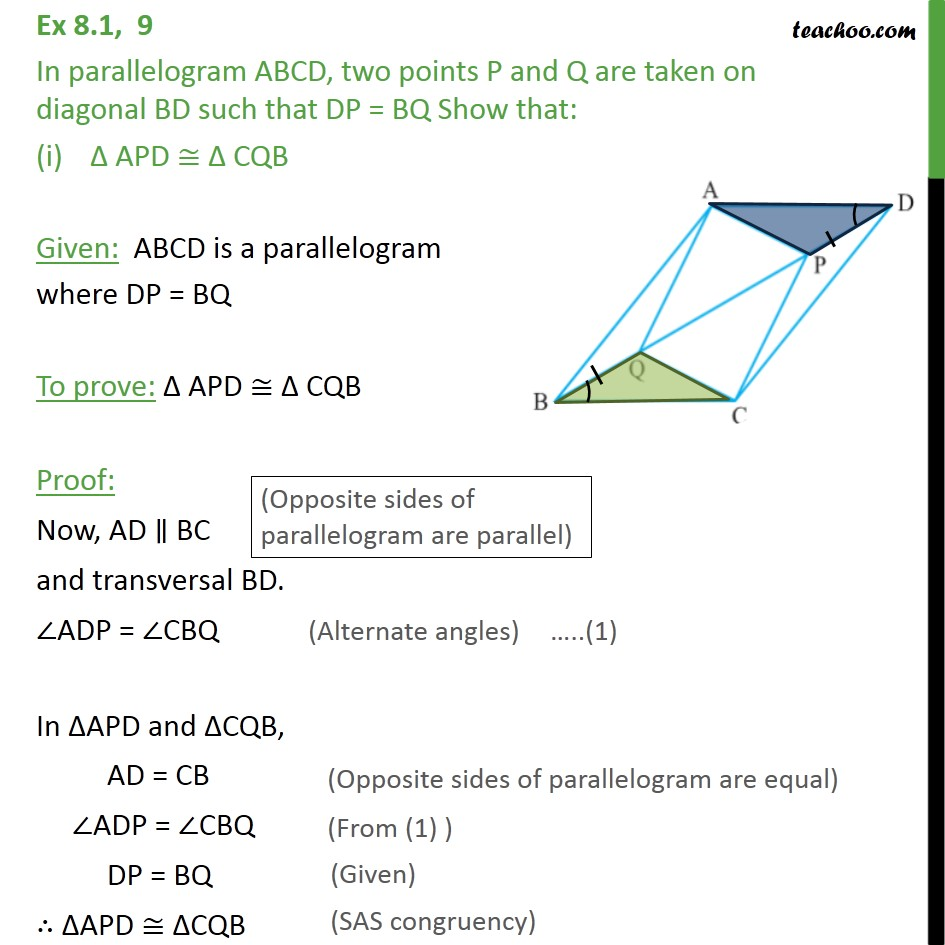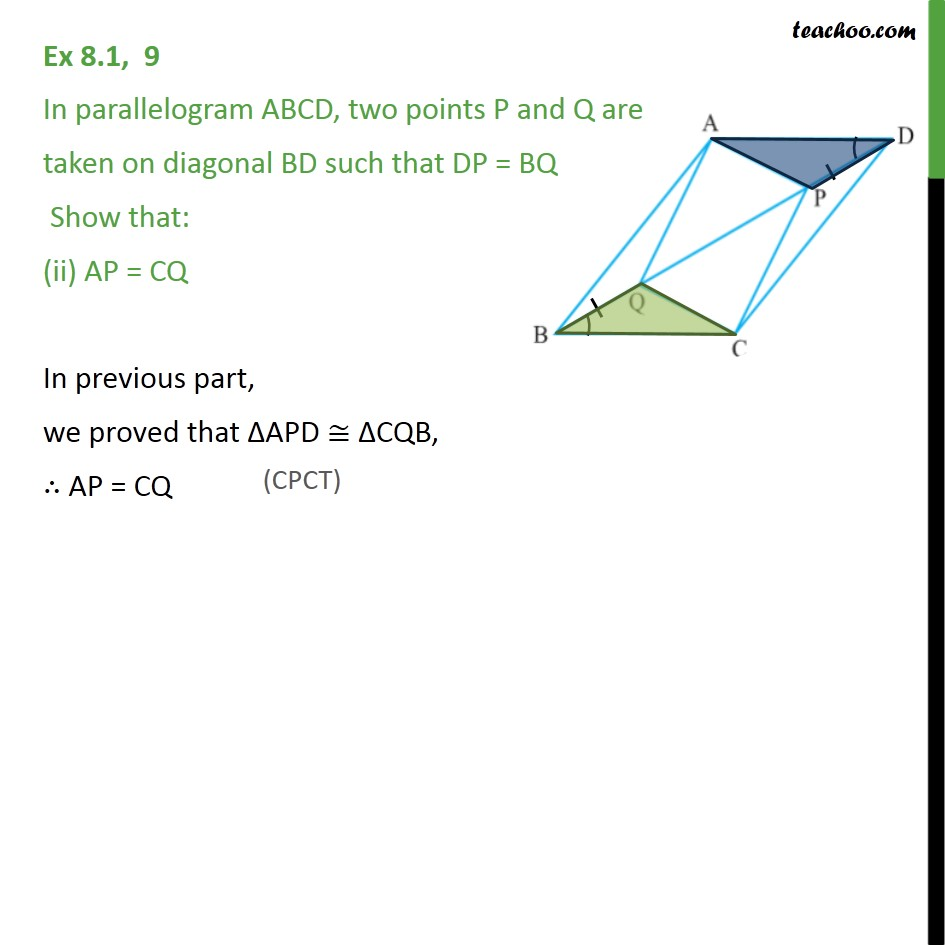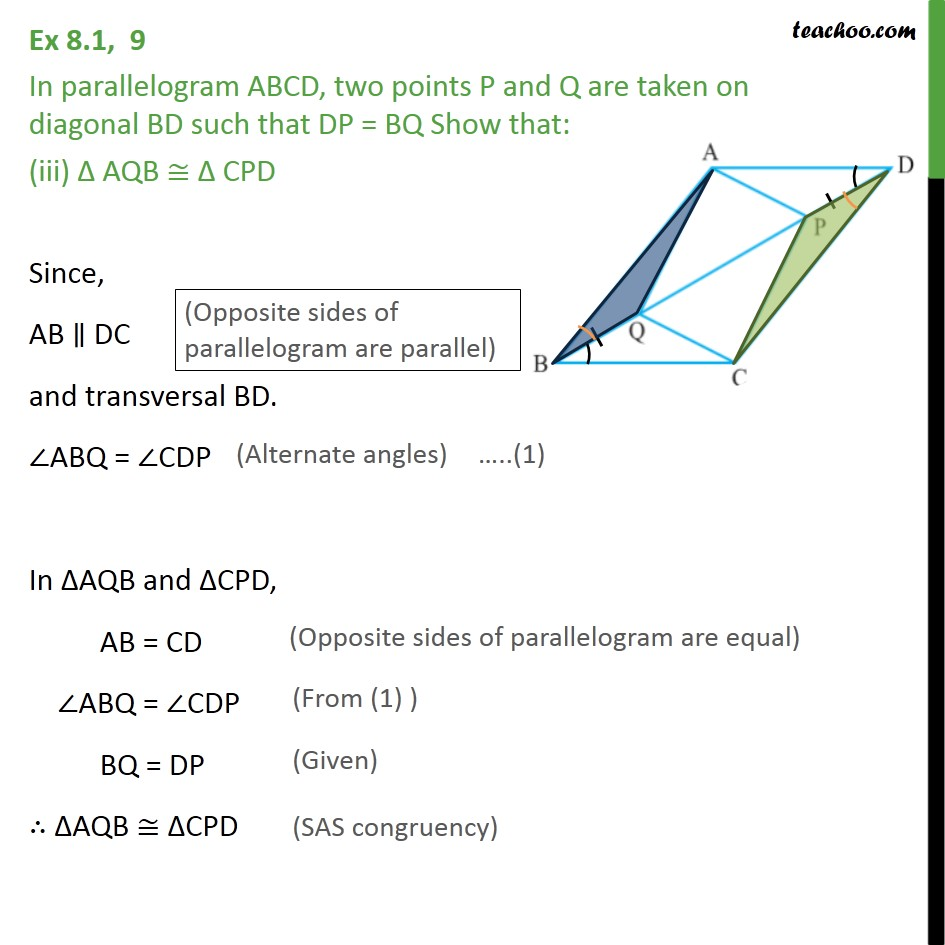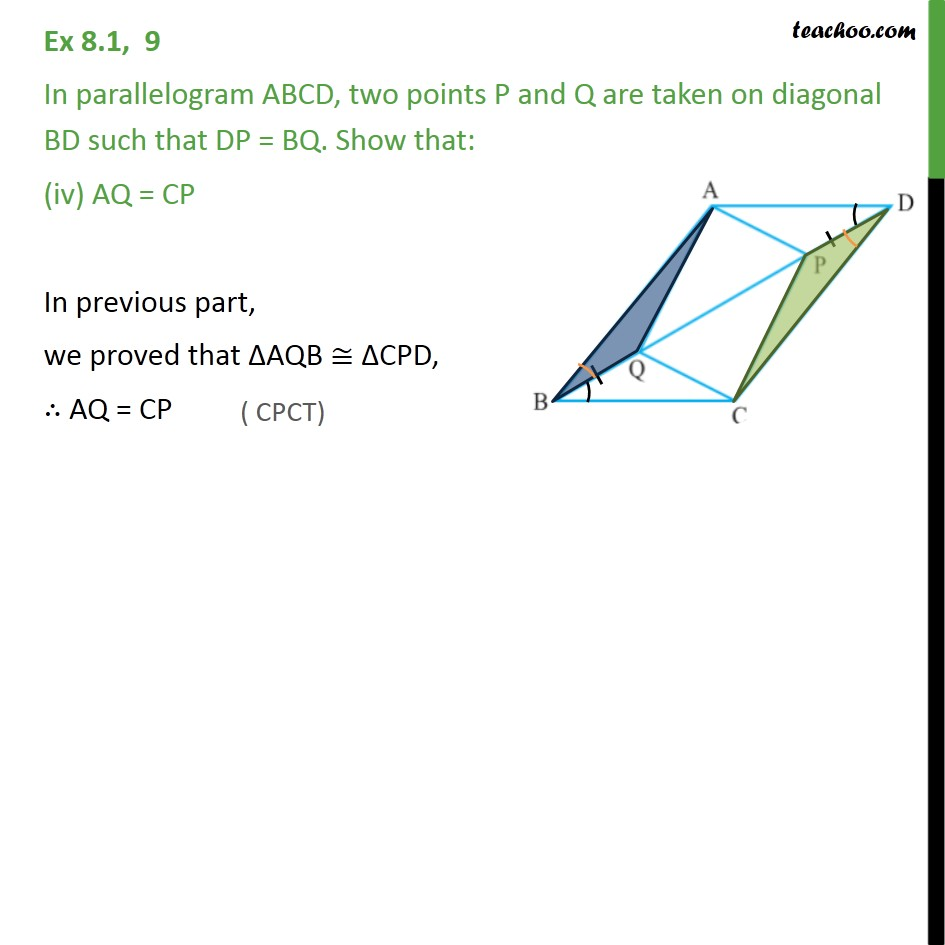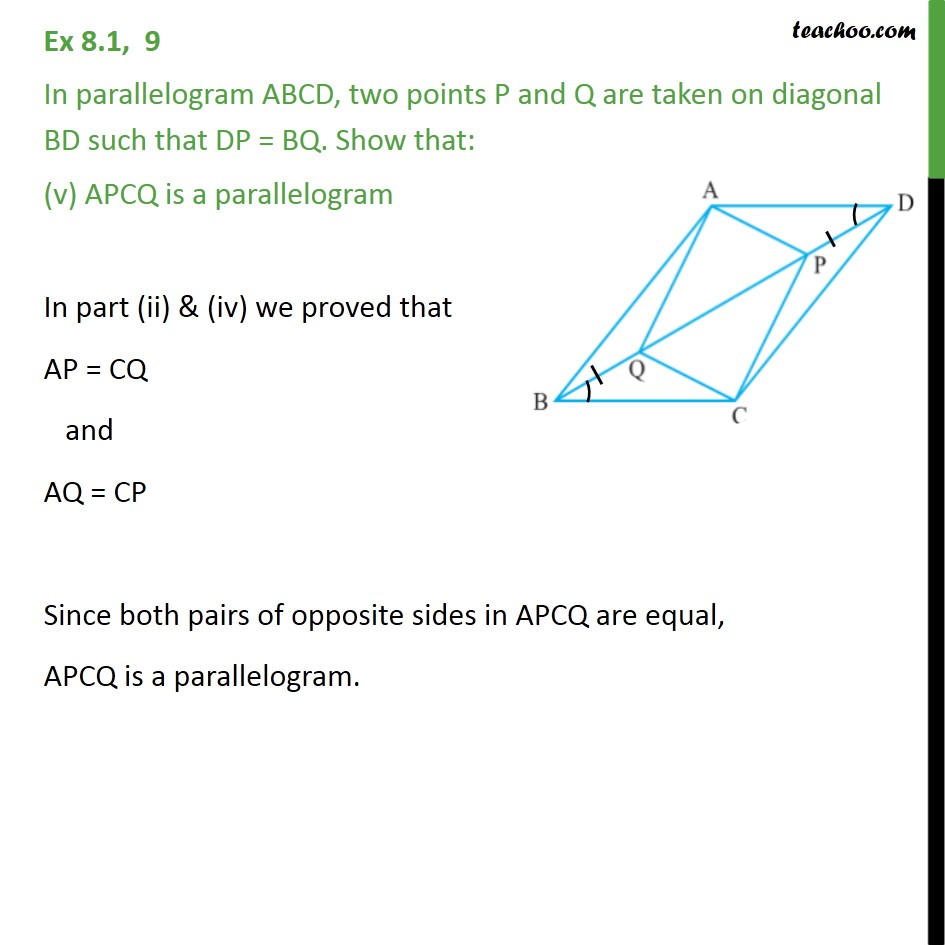Learn in your speed, with individual attention - Teachoo Maths 1-on-1 Class

### Transcript

Ex 8.1, 9 In parallelogram ABCD, two points P and Q are taken on diagonal BD such that DP = BQ Show that: APD CQB Given: ABCD is a parallelogram where DP = BQ To prove: APD CQB Proof: Now, AD BC and transversal BD. ADP = CBQ In APD and CQB, AD = CB ADP = CBQ DP = BQ APD CQB Ex 8.1, 9 In parallelogram ABCD, two points P and Q are taken on diagonal BD such that DP = BQ Show that: (ii) AP = CQ In previous part, we proved that APD CQB, AP = CQ Ex 8.1, 9 In parallelogram ABCD, two points P and Q are taken on diagonal BD such that DP = BQ Show that: (iii) AQB CPD Since, AB DC and transversal BD. ABQ = CDP In AQB and CPD, AB = CD ABQ = CDP BQ = DP AQB CPD Ex 8.1, 9 In parallelogram ABCD, two points P and Q are taken on diagonal BD such that DP = BQ. Show that: (iv) AQ = CP In previous part, we proved that AQB CPD, AQ = CP Ex 8.1, 9 In parallelogram ABCD, two points P and Q are taken on diagonal BD such that DP = BQ. Show that: (v) APCQ is a parallelogram In part (ii) & (iv) we proved that AP = CQ and AQ = CP Since both pairs of opposite sides in APCQ are equal, APCQ is a parallelogram.